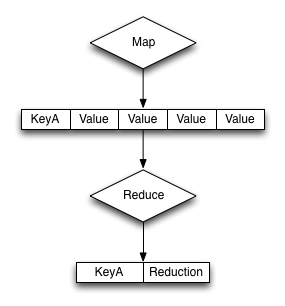# Custom Reduce Functions

• concept
+
The `reduce()` function has to work slightly differently to the `map()` function.

In the primary form, a `reduce()` function must convert the data supplied to it from the corresponding `map()` function.

The core structure of the reduce function execution is shown the figure below.The base format of the `reduce()` function is as follows:

```function(key, values, rereduce) {
…

return retval;
}```

The reduce function is supplied three arguments:

• `key`

The `key` is the unique key derived from the `map()` function and the `group_level` parameter.

• `values`

The `values` argument is an array of all of the values that match a particular key. For example, if the same key is output three times, `data` will be an array of three items containing, with each item containing the value output by the `emit()` function.

• `rereduce`

The `rereduce` indicates whether the function is being called as part of a re-reduce, that is, the reduce function being called again to further reduce the input data.

When `rereduce` is false:

```* The supplied `key` argument will be an array where the first argument is the
`key` as emitted by the map function, and the `id` is the document ID that
generated the key.

* The values is an array of values where each element of the array matches the
corresponding element within the array of `keys`.```

When `rereduce` is true:

```* `key` will be null.

* `values` will be an array of values as returned by a previous `reduce()`
function.```

The function should return the reduced version of the information by calling the `return()` function. The format of the return value should match the format required for the specified key.

Output